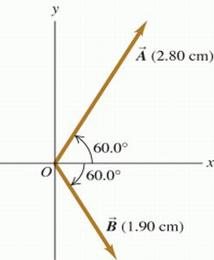# Question Solved1 AnswerPart A For the two vectors in the figure (Figure 1) , find the magnitude of the vector product A⃗ ×B⃗ . Part B Find the direction of the vector product A⃗ ×B⃗ . Find the direction of the vector product . +z-directionPart A

For the two vectors in the figure (Figure 1) , find the magnitude of the vector product A⃗ ×B⃗ .

Part B

Find the direction of the vector product A⃗ ×B⃗ .

Find the direction of the vector product .

 +z-direction or -z-direction Part C Find the magnitude of B⃗ ×A⃗ .

Part D

Find the direction of B⃗ ×A⃗ .

 +z-direction  or -z-direction

Transcribed Image Text: Part A For the two vectors in the figure (Figure 1) , find the magnitude of the vector product A⃗ ×B⃗ . Part B Find the direction of the vector product A⃗ ×B⃗ . Find the direction of the vector product . +z-direction
More
Transcribed Image Text: Part A For the two vectors in the figure (Figure 1) , find the magnitude of the vector product A⃗ ×B⃗ . Part B Find the direction of the vector product A⃗ ×B⃗ . Find the direction of the vector product . +z-direction